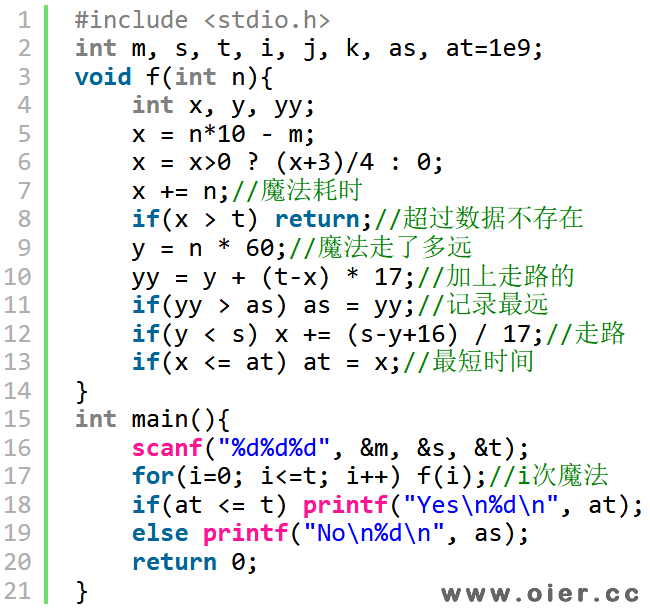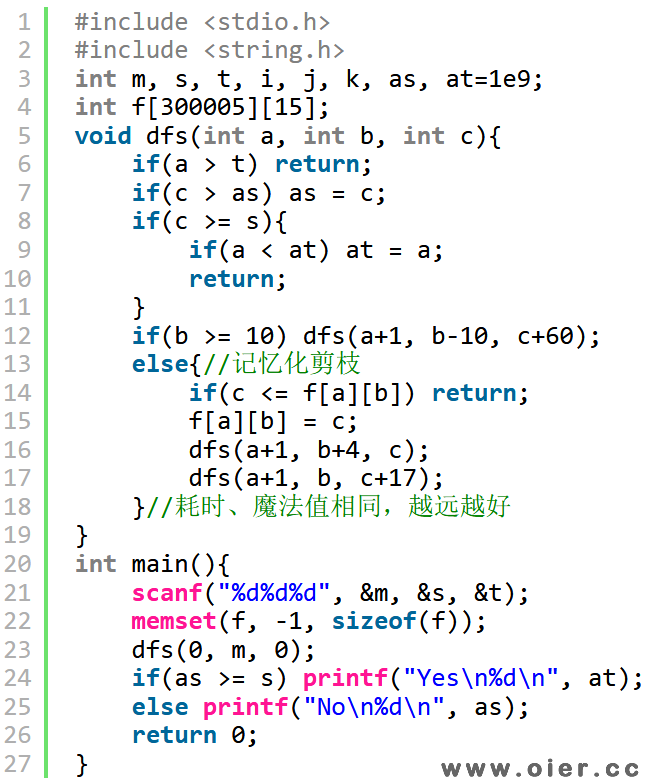SSOJ1278守望者的逃离（NOIP2007）
1447+

【输入样例1】
39 200 4

【输入样例2】
36 255 10

【输出样例1】
No
197

【输出样例2】
Yes
6

提示

30%的数据满足：1< =T< =10,1< =S< =100

50%的数据满足：1< =T< =1000,1< =S< =10000

100%的数据满足：1< =T< =300000,0< =M< =1000,1< =S< =108

NOIP2007普及组第三题

程序实现